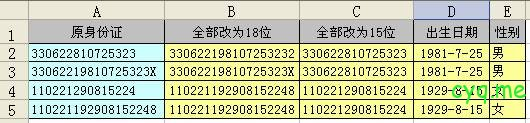## 15位身份证改18位，最后一位的计算

15位身份证改为18位，是在原第6位后插入19,然后再补上最后一位生成18位的。

（1）十七位数字本体码加权求和公式

S = Sum(Ai * Wi), i = 0, … , 16 ，先对前17位数字的权求和

Ai:表示第i位置上的身份证号码数字值

Wi:表示第i位置上的加权因子

Wi: 7  9  10  5  8  4  2  1  6  3  7  9  10  5  8  4  2

（2）计算模

Y = mod(S, 11)

（3）通过模得到对应的校验码

Y: 0 1 2 3 4 5 6 7 8 9 10

excel的身份证转换公式：

1、身份证号全部改为18位，输入数组公式：

=IF(LEN(A2)=15,REPLACE(A2,7,,19)&MID(“10X98765432”,MOD(SUM(MID(REPLACE(A2,7,,19),ROW(INDIRECT(“1:17”)),1)*2^(18-ROW(INDIRECT(“1:17”)))),11)+1,1),A2)

2、身份证号全部改为15位，输入公式：

=IF(LEN(A2)=15,A2,LEFT(REPLACE(A2,7,2,),15))

3、计算出生日期：

=IF(A2<>””,TEXT((LEN(A2)=15)*19&MID(A2,7,6+(LEN(A2)=18)*2),”#-00-00″)+0,)

4、判断性别：

=IF(A2<>””,IF(MOD(RIGHT(LEFT(A2,17)),2),”男”,”女”),)